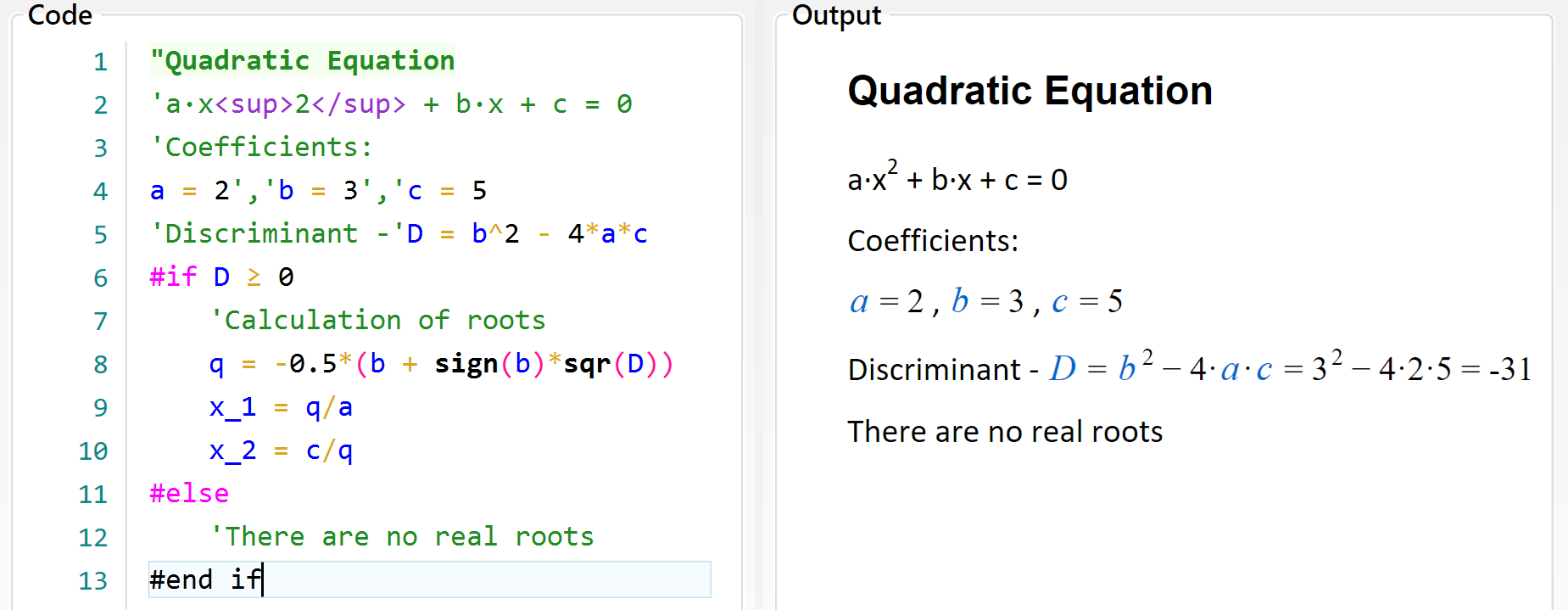Topic: Reporting

## Conditional execution

Sometimes the solution have to continue in different ways, depending on some intermediate values. Such feature is included in Calcpad, similarly to other programming languages. It is called "conditional execution block" and has the following general form:

• `#If condition1`
• contents if condition1 is satisfied
• `#Else If condition2`
• contents if condition2 is satisfied
• `#Else If condition3`
• contents if condition3 is satisfied
• . . .
• `#Else`
• contents if none of the conditions is satisfied
• `#end if`

Shorter forms are also possible:

• `#If condition`
• contents if the condition is satisfied
• `#Else`
• contents if the condition is not satisfied
• `#end if`

or:

• `#If condition`
• contents if the condition is satisfied
• `#end if`

Condition blocks affect not only the calculation path but also the report content like text and images. The "#" symbol must be the first one in the line. At the place of "condition" you can put any valid expression. Normally, a comparison is used like "#If a < 0", but it is not obligatory. If it evaluates to any nonzero number, the condition is assumed to be satisfied. Otherwise, it is not satisfied. Any result which absolute value is ≤ 0.00000001 is assumed to be zero.

Let us look again at the quadratic equation example that we used earlier. If we enter "c = 5", the discriminant will be negative and the result will be NaN. This is not a very intelligent way to finish a program. What we need to do is to check if "D < 0" and if so, to provide a comprehensible message. Otherwise, we have to calculate the roots. We can do this, using conditional execution, as follows: